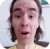FreeKB - Perl Date and time using Time::Piece
Perl - Date and time using Time::PieceUse the Time::Piece module.

``````#!/usr/bin/perl

use warnings;
use strict;
use Time::Piece;

my \$time = Time::Piece->new;
my \$datetime = \$time->strftime("%b %d, %Y");

print "\$datetime\n";``````

This will produce a result in this format.

``Jan 16, 2019``

Following are commonly used options.

Option Description Example
%Y Year 2018
%y Year 18
%m Month 01
%b Month Jan
%B Month January
%d Day (with leading zero) 04
%-d Day (without leading zero) 4
%H Hour (24 hour format) 22
%I Hour (12 hour format) 10
%M Minute 56
%S Second 23
%p AM or PM AM
%P am or pm pm

Future / Past date

The add_years option can be used to add or subtract years. This will go back 1 year.

``my \$lastYear = \$time->add_years(-1)->strftime(%b %d, %Y);``

However, consider it is January.

If you use add_months(-1) then it becomes December, and you would also have to include add_years(-1). This can get unruley quickly. There is where Time::Seconds comes in handy.

``````#!/usr/bin/perl

use strict;
use warnings;
use Time::Piece;
use Time::Seconds;

my \$time           = Time::Piece->new;
my \$one_month_forward  = (\$time + ONE_MONTH)->strftime("%Y%m%d");
my \$two_month_forward  = (\$time + ONE_MONTH * 2)->strftime("%Y%m%d");
my \$one_month_backward = (\$time - ONE_MONTH)->strftime("%Y%m%d");
my \$two_month_backward = (\$time - ONE_MONTH * 2)->strftime("%Y%m%d");
my \$one_day_forward    = (\$time + ONE_DAY)->strftime("%Y%m%d");
my \$one_day_backward   = (\$time - ONE_DAY)->strftime("%Y%m%d");
my \$two_day_backward   = (\$time - ONE_DAY * 2)->strftime("%Y%m%d");

print "\\$two_month_backward = \$two_month_backward \n";
print "\\$one_month_backward = \$one_month_backward \n";
print "\\$one_month_forward  = \$one_month_forward \n";
print "\\$two_month_forward  = \$two_month_forward \n";
print "\\$two_day_backward   = \$two_day_backward \n";
print "\\$one_day_backward   = \$one_day_backward \n";
print "\\$one_day_forward    = \$one_day_forward \n";``````

We will never share your name or email with anyone. Enter your email if you would like to be notified when we respond to your comment.

Please enter fce35 in the box below so that we can be sure you are a human.

Web design by yours truely - me, myself, and I   |   jeremy.canfield@freekb.net   |